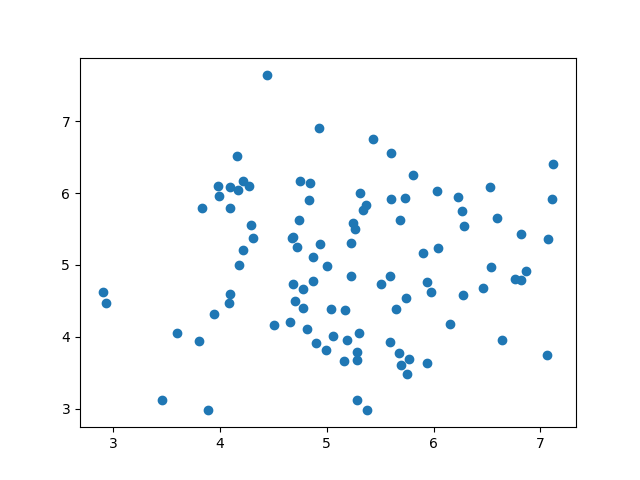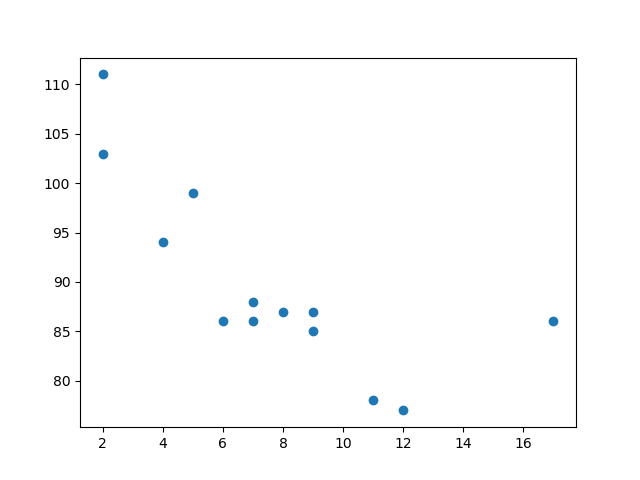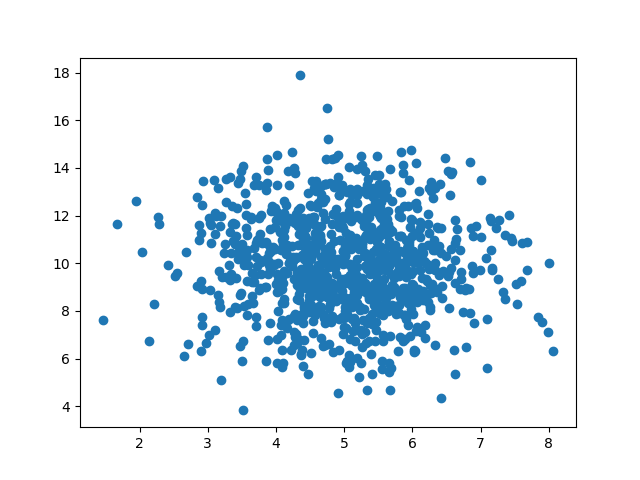# 机器学习 - 散点图

## 散点图（Scatter Plot）Matplotlib 模块有一种绘制散点图的方法，它需要两个长度相同的数组，一个数组用于 x 轴的值，另一个数组用于 y 轴的值：

```x = [5,7,8,7,2,17,2,9,4,11,12,9,6]

y = [99,86,87,88,111,86,103,87,94,78,77,85,86]
```

x 数组代表每辆汽车的年龄。

y 数组表示每个汽车的速度。

### 实例

```import matplotlib.pyplot as plt

x = [5,7,8,7,2,17,2,9,4,11,12,9,6]
y = [99,86,87,88,111,86,103,87,94,78,77,85,86]

plt.scatter(x, y)
plt.show()
```

### 结果：x 轴表示车龄，y 轴表示速度。

## 随机数据分布

### 实例

```import numpy
import matplotlib.pyplot as plt

x = numpy.random.normal(5.0, 1.0, 1000)
y = numpy.random.normal(10.0, 2.0, 1000)

plt.scatter(x, y)
plt.show()
```

### 结果：